AP State Board Syllabus AP SSC 10th Class Physical Science Important Questions Chapter 4 Acids, Bases and Salts.

## AP State Syllabus SSC 10th Class Chemistry Important Questions 4th Acids, Bases and Salts

### 10th Class Chemistry 4th Lesson Acids, Bases and Salts 1 Mark Important Questions and Answers

Question 1.
Take some water in a test tube and add concentrated H2SO4 to it. Shake the test tube well. If you touch the bottom of the test tube, you feel it as hot. Now, instead of H2SO4, if you add NaOH pellets to water in another test tube and touch the bottom, what do you observe? (TS June 2015)
The bottom of test tube is also hot because reactions of acids, bases with water are exothermic reactions.

Question 2.
What happens if the copper sulphate crystals taken into dry test tube are heated? (TS June 2016)

• When copper sulphate crystals are heated, water present in crystals is evaporated and the salt turns white.
• Evaporated water appears as droplets on the walls of the test tube.
• Blue coloured copper sulphate (CuSO4 5H2O) is turned into white colour because 5H20 molecules are evaporated from crystals.Question 3.
Why does the soil of agricultural lands get tested for pH?
Plants require a specific pH range for their healthy growth. So, finding pH of a soil suggested the farmers to treat the fields with acidic or basic substances to maintain the required pH range.

Question 4.
Write the molecular formulae of common salt and baking soda which are widely used at home. (TS June 2017)
Common Salt: NaCl; Backing soda : NaHCO3

Question 5.
Mention the precautions to take while conducting an experiment to prove acids produce ions only in aqueous solutions. (TS June 2018)

• Testing of the evolved gas by using dry litmus paper first. Then with wet litmus paper.
• Use gaurd tube containing calcium chloride.

Question 6.
What are antacids?
Antacids are mild alkalies. These are used for getting relief from acidity and indigestion and sometimes even headache. When taken orally, it reacts with hydrochloric acid present in the stomach and reduces its strength by consuming some of it.
Ex: Milk of magnesia.Question 7.
Tap water conducts electricity whereas distilled water does not. Why?
Tap water contains some impurities in the form of salts. Due to presence of salts, it conducts electricity. Distilled water is free from all kinds of salts and hence does not conduct electricity.

Question 8.
What do you mean by dilution of an acid or base? Why is it done?
Dilution of an acid or base means mixing an acid or base with water. This is done to decrease the concentration of ions per unit volume. In this way the acid or the base is said to be diluted.

Question 9.
What is a universal indicator?
An indicator which passes through a series of colour changes over a wide range of H3O+ ions concentration is called universal indicator.

Question 10
What is tooth decay?
Tooth enamel is chemically calcium phosphate Ca3(PO4)2. It starts corroding when pH falls below 5.5. Food particles left in the mouth degrade to produce acid which lower the pH of the mouth. This is called tooth decay.

Question 11.
Define Alkalis and give some examples.
Alkalis : An alkali is a base that dissolves in water.

Examples :
i) Sodium Hydroxide (NaOH),
ii) Potassium Hydroxide (KOH),
iii) Magnesium Hydroxide (Mg(OH)2)

Question 12.
Why should we not taste or touch alkalis?
We should not taste or touch alkali. Because they are corrosive.

Question 13.
Salts conduct electricity. Why?
Salts contains ions. So they conduct electricity.

Question 14.
Why are calcium sulphate hemihydrates called Plaster of Paris?
Calcium sulphate hemihydrates are used as plaster for supporting fractured bones in the right position. So, it is called Plaster of Paris.

Question 15.
Why does an aqueous solution of acid conduct electricity?
An aqueous solution of acid liberates H+ ions. This makes the aqueous solution of acid to conduct electricity.

Question 16.
How is the concentration of hydronium ions (H3O+) affected when a solution of an acid is diluted?
When a solution of an acid is diluted, concentration of H3O+ ions decreases.Question 17.
What is pH?
A. pH is a scale for measuring hydrogen ion concentration in a solution. It is the negative logarithm of H+ concentration.
pH = – log [H+].

Question 18.
How is pH of a solution related to the [H3O+] of that solution?
The presence of H3O+ ions indicate us whether it is a strong acid or weak acid.

Question 19.
There are two solutions of pH values 6 and 8. Which solution has more hydrogen ion concentration? Which of this is acidic and which one is basic?

• The solution whose pH value 6 is acid and has more hydrogen ion concentration.
• The solution of pH value 8 is basic and has less hydrogen ion concentration.

Question 20.
Can you give example for use of olfactory indicators in daily life?
Examples of olfactory indicators : Onion, vanilla extract.

Question 21.
How do acids neutralize bases?
(OR)
How do acids and bases react with each other?
According to Arrhenius theory acids produce H+ ions and bases produce OH ions in aqueous media.
The combination of H+ and OH ions is called ‘neutralization’.
Thus acids neutralize bases.

Question 22.
How strong are acids and base solutions?
The acids of pH value as much less as possible have more concentration [pH < 7], Basic nature increases as pH value increases.

Question 23.
What do you say about salts of both weak acid and weak base?
The pH of aqueous solutions of salt obtained from both weak acid and weak base is nearly 7.

Question 24.
Which base is used for removing permanent hardness of water?
Sodium carbonate is used for removing permanent hardness of water.

Question 25.
Name two antacids used to get rid of our indigestion problem.
Magnesium hydroxide and a mild base (baking soda).

Question 26.
Under what soil conditions would a farmer would treat the soil of his fields with quicklime (calcium hydroxide) or calcium carbonate?
When the field has acidic nature, the farmer uses quicklime or calcium carbonate to neutralize it.

Question 27.
Write the formulas of Gypsum and Plaster of Paris.
The formulae of Gypsum is CaSO4 . 2H2O and Plaster of Paris is CaSO4 . ½H2O.Question 28.
Write any two Acid Base indicators.

1. Methyl orange
2. Phenolphthalein.

Question 29.
Which salt is used in the manufacture of borax?
Washing soda (Na2CO3.10H2O)

Question 30.
What is family of salts? Give examples.
Salt having the same positive or negative radical is called family of salts.
Eg : Family of sodium salts : NaCl, Na2SO4
Family of chloride salts : NaCl, KCl.

Question 31.
‘A’ is a substance which is acidic and it is added in solution to preserve pickles. What is A and what is the name given to its dilute solution?
‘A’ is acetic acid and its dilute solution is called vinegar.

Question 32.
What are the chemical names of the following?
1) Baking soda
2) Gypsum

• The chemical name of baking soda is sodium hydrogen carbonate (NaHCO3).
• The chemical name of gypsum is calcium sulphate dihydrate (CaSO4 • 2 H2O).

Question 33.
Write the water of crystallisation of following compound.
a) Hydrous copper sulphate
b) Washing soda
c) Gypsum
d) Plaster of Paris

 Compound Formula Water of crystallisation 1) Hydrous copper sulphate CuSO4 . 5H2O 5 2) Washing soda Na2CO3 . 10 H2O 10 3) Gypsum CaSO4 . 2 H2O 2 4) Plaster of Paris CaSO4 . ½H2O ½

Question 34.
Why don’t we use a strong base like NaOH as antacid?
Strong bases like potassium hydroxide (KOH), sodium hydroxide (NaOH) are corrosive in nature. So they can harm the internal organs. Therefore we should not use them as antacid.

Question 35.
What do you mean by HsO+ ion?
Hydrogen ions cannot exist as base ions. They associate with water molecules and exist as hydrated ions with each H+ attached by 4 to 6 water molecules. For this we represent H+ as hydronium ion, H3O+.
H+ + H2O → H3O+

Question 36.
Which indicator is useful at all pH? Why?
Universal indicator is useful to test solutions of all pH because it gives different colours at different pH range.

Question 37.
Which substance is useful in removing permanent hardness of water?
The permanent hardness of water is due to chloride and sulphate salts of magnesium and calcium, which can be removed by adding washing soda.

Question 38.
Given two examples for strongest bases.
Sodium hydroxide – NaOH
Potassium hydroxide – KOHQuestion 39.
What is the confirmation test for hydrous and anhydrous salt?

• On heating hydrous salt in a test tube it will form water droplets on the sides of test tube.
• On heating anhydrous salt in a test tube it will not form water droplets on the sides of test tube.

Question 40.
P.O.P, cement, calcium chloride should be stored in moisture proof containers. Why?

• P.O.P, cement and calcium chloride react with moisture (H2O) in the atmosphere and set into hard solid masses.
• To avoid availability of moisture they should be stored in moisture proof containers.

Question 41.
Give some examples for hydrous and anhydrous salts.
Eg : For hydrous salts :

1. CuSO4 . 5H2O
2. Na2CO3 . 10H2O
3. CaSO4 . 2H2O

Eg : for anhydrous salts :

1. NaCl
2. MgCl2
3. Na2CO3

Question 42.
How are bitter and sour taste substances tested without testing?

• Sour taste substances turn blue litmus to red.
• Bitter taste substances turn red litmus to blue. By these tests we can test them as acids and bases.

Question 43.
Do the metallic oxides react with acids?
Yes, metallic oxides are basic in nature. They react with acids and form salt and water.

Question 44.
Does non-metallic oxide react with base?
Yes, non-metallic oxide is acidic in nature. It reacts with base and forms salt and water.

Question 45.
Why does dry HCl gas not change the colour of the dry litmus paper?
(OR)
Prove that dry HCl gas is not an acid but HCl aqueous solution is an acid using an activity.

• Dry hydrogen chloride gas is not an acid. Hence it can’t turn blue litmus into red.
• Hydrochloric acid is an aqueous solution. Hence it can turn blue litmus into red.

Question 46.
Do you know that the atmosphere of Venus is made up of thick white and yellowish clouds of sulphuric acid? Do you think life can exist on this planet?

1. No, it is not possible.
2. When pH value decreases, the survival of living organisms becomes difficult.
3. Hence there is not any possibility of life on Venus.

Question 47.
Why do acids not show acidic behaviour in the absence of water?
Acids don’t show acidic behaviour in the absence of water as H+ ions are absent in them.

Question 48.
How is the concentration of hydroxide ions (OH) affected when excess base is dissolved in a solution of sodium hydroxide?
When a base like NaOH (Sodium hydroxide) is dissolved in water, it liberates (OH) ions.
Equation :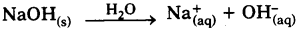OH ion concentration increases.

Question 49.
How does the nature of the solution change with change in concentration of H+(aq) ions?
The concentration of H+ ions is responsible for the acidic nature of a substance.
If [H+] > 1 × 10-7 mol/lit the solution is acidic.
If [H+] < 1.0 × 10-7 mol/lit the solution is basic.

Question 50.
Do basic solutions also have H+(aq) ions? If yes, then why are these basic?
Yes. Basic solutions have OH(aq) ions and bases have less number of H3O+ ions. H+(aq) ions are less in base. In basic solution [OH] > [H+].

Question 51.
What do acids have in common?
(Acids have sour taste and conduct electricity. They release H2 (Hydrogen) gas on reacting with metals). All acids have H+(aq) ions.

Question 52.
What do bases have in common?
Bases are slippery to touch and bitter to taste. All bases have OH(aq) ions.

Question 53.
Why does pure acetic acid not turn blue litmus to red?
Pure acetic acid is a weak acid so it does not have sufficient H+(aq) ion to change the colour of blue litmus to red.

Question 54.
What will happen if the pH value in your body increases?
It affects our digestion system.

Question 55.
A student checked pH of a salt solution and found that its pH is more than 7. How is that type of salt formed?

• When a strong base reacts with weak acid then the solution is basic in nature. So its pH is more than 7.
• For example when acetic acid reacts with sodium hydroxide the salt formed has basic nature.Question 56.
Explain the procedure that you follow to reduce water from a given salt.
Procedure to reduce water from a given salt :

1. Take a boiling test tube.
2. Drop given salt in the test tube.
3. Heat the test tube gently.
4. Water from salt evaporates.
5. In this way we can reduce the water from salt.

Question 57.
Write the observations, when the hydrated salt or unhydrated salt is heated.

• When hydrated salt is heated water droplets form inside the walls of test tube and sometimes blue or green colour salt turns into white colour.
• When unhydrated salt is heated it does not form water droplets inside the test tube walls and colour also does not change.

Question 58.
On heating the hydrated salt it loses water molecules present in it. To show this what are the equipment required?
1) On heating the hydrated salt it loses water molecules present in it.
2) To show this the given equipment are required

1. Boiling tube
2. Test tube holder
3. Burner

Question 59.
Try to collect the information to reasons for calling calcium sulphate hemihydrates as Plaster of Paris (POP).

• Gypsum plaster (or) Plaster of Paris (POP) is produced by heating gypsum to about 300°F.
• A large gypsum deposit is found at Montmartre in Paris (France).
• This gave the name Plaster of Paris to calcium sulphate hemihydrates.
• The term plaster can refer to gypsum.

Question 60.
Is the substance present in antacid tablet acidic or basis?
The substance present antacid is weakly basic.

Question 61.
Give pH of neutral, acid and base.

 Nature of substance pH range Neutral 7 Acid 0 – 7 Base 7 – 14

Question 62.
Which nature of Plaster of Paris makes its importance? Appreciate it.
Plaster of Paris is a white powder. It is very soft and can be used to make toys, materials for decoration and to make surfaces smooth.

But on mixing with water, it changes to a hard solid mass (Gypsum). This is the important character of Plaster of Paris (POP).

Question 63.
What is acid rain? How does it affect our aquatic life?
When the pH of rain water is less than 5.6 it is called acid rain. When acid rain flows into the rivers, it lowers the pH of the river water. Since our body works within a narrow pH range close to 7 the survival of aquatic life in river water mixed with rain water becomes difficult.

Question 64.
Why are pickles and sour substances not kept in brass and copper vessels?
Pickles and sour substances contain acidic nature which may react with brass and copper vessels to produce toxic substances.
So, we don’t keep them in brass arid copper vessels.Question 65.
Can you suggest some examples of use of pH in everyday life?
Uses of pH in everyday life :

1. pH value helps us to identify acids, bases and neutrals.
2. If pH value is less in our mouth, it leads to tooth decay. We can find it as the reason for our tooth decay.
3. pH value helps us to know about acid rain.

Question 66.
Write any two uses of Bleaching powder.

• It is used for disinfecting drinking water to make it free of germs.
• It is used as a reagent in the preparation of chloroform.

### 10th Class Chemistry 4th Lesson Acids, Bases and Salts 2 Marks Important Questions and Answers

Question 1.
What value of pH in the mouth leads to tooth decay? Why? (TS June 2015)

• Tooth decay starts when the pH of the mouth is lower than 5.5.
• Tooth enamel, made of calcium phosphate is the hardest substance in the body.
• It does not dissolve in water, but is corroded when the pH in the mouth is below 5.5.
• Bacteria present in the mouth produce acids by degradation of sugar and food particles remaining in the mouth.

Preventions :

1. Clean the mouth after eating food.
2. Using tooth pastes, which are generally basic neutralize the excess acid and pre¬vent tooth decay.

Question 2.
Equal lengths of Magnesium ribbons are taken in two test-tubes X and Y. Hydro¬chloric acid is added to test-tube X and Acetic acid is added to test-tube Y. In which test-tube, the reaction will be more vigorous? Why? (TS March 2015)
The speed of the reactions is higher in X test tube than Y test tube.

Reason :
Due to strong acidic nature, Hydrochloric acid reacts very fast with magnesium ribbon.

Question 3.
Name the four chemicals that are obtained from common salt and write their molecular formulae. (TS March 2015)
Chemicals that can be obtained from common salt are

1. Sodium Hydroxide – NaOH
2. Baking soda / Cooking soda / Caustic soda / Sodium bicarbonate / Sodium Hydrogen carbonate. – NaHCOv
3. Washing soda / Sodium carbonate – Na2CO3 10H2O
4. Bleaching powder / Calcium Oxy Chloride – CaOCl2

Question 4.
Observe the information given in the table and answer the questions given below the table. (TS March 2017)

 Substance (in aqueous solution) Colour change with Blue Litmus Colour change with Red Litmus A Red No change B No change Blue C No change No change

i) Which one of them may be the neutral salt among A, B, C?
ii) What may happen when some drops of phenolphthalein is added to the substance B?
i) C
ii) Pink Colour

Question 5.
Why do we use antacids? Write it’s nature. (TS March 2018)
Pain and irritation will be caused in stomach during the acidity problem/indigestion problem. Antacids used to neutralize the excess acid in the stomach and gives relief from acidity Antacids are basic in nature.

Question 6.
Which product will form when CaO is dissolved in water? How do you find the nature of product? (TS March 2018)
CaO reacts with water and gives calcium hydroxide [Ca(OH2)]. The nature of the calcium hydroxide will be tested with red litmus paper or pH paper.

Calcium Hydroxide turns red litmus into blue. Thus we can say that ca(OH)2 is basic in nature.

Ca(OH)2 shows pH value more than 7. Thus we can say that Ca(OH)2 is basic in nature.Question 7.
How do you test the nature of the solution formed by dissolving CaO in water? What is the nature of the solution? (TS June 2019)

• The solution formed by dissolving CaO in water is tested with red litmus paper, it turns into blue colour, (or) It is tested with methyl orange, it turns into yellow in colour.
• The solution of CaO and water is basic in nature.

Question 8.
Write the experimental procedure to test carbon dioxide gas. (AP SCERT: 2019-20)

1. Pass the CO2 gas through lime water [Ca(OH)2].
2. The lime water appears as milky white.
3. The reaction is Ca(OH)2 + CO2 → CaCO3 ↓ + H2O.
4. The milky white is caused by CaCO3.

Question 9.
Write two reactions of acids with carbonates and metal hydrogen carbonates. (AP SA-I : 2019-20)
Reactions :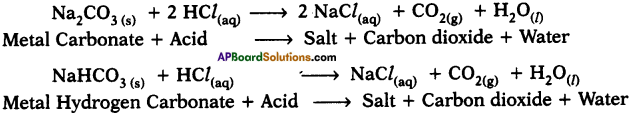Question 10.
What do acids have in common?
Common characteristics of acids :

1. Similar chemical properties.
2. Acids generate hydrogen gas on reacting with metals.
3. Hydrogen is common to acids.
4. Acids are sour in taste and turn blue to red when react with bases form salt and water.

Question 11.
What do bases have in common?
Common characteristics of bases :

1. Bitter in taste.
2. Soapy in nature.
3. Turn red to blue colour.
4. On heating decompose into metal oxides and water.
5. React with acids to form salt and water.
6. Produce OH ions in aqueous solution.

Question 12.
How is bleaching powder produced?
Production of bleaching powder :
Bleaching powder is produced by the action of chlorine on dry slaked lime (Ca(OH)2).
Equation :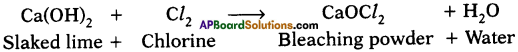Question 13.
What can you conclude about the ideal soil pH for the growth of plants in your region?
1) Soil is considered the ‘skin of the earth’. The soil pH plays a vital role in the growth of a plant and it influences plant nutrition.
2) Soil pH strongly affects the nutrients required for the plant growth.
3) The nutrients may be stored on soil colloids, and live or dead organic matter, but may not be accessible to plants due to extremes of pH.

Conclusion :
For optimum plant growth, the generalized content of soil components by volume should be roughly 50% solids (45% mineral & 5% organic matter), and 50% voids of which half is occupied by water and half by gas.

Question 14.
How can you prepare turmeric indicator? What is the use of it?
i) Turmeric indicator is prepared from turmeric.
ii) It has red colour in basic solution.Question 15.
Name two salts and write their formulae which possess water of crystallization.

1. Hydrous copper sulphate. Its formula is CuSO4 . 5H2O
2. Gypsum. Its formula is CaSO4 . 2H2O

Question 16.
What is neutralization reaction? Give two examples.
When an acid reacts with base it forms salt and water. This reaction is called neutralisation reaction.
e.g.: NaOH(aq) + HCl(aq) → NaCl(aq) + H2O(l)
H2SO4(aq) + 2Na0H(aq) → Na2SO4(aq) + 2H2O(l)

Question 17.
All alkalis are bases but all bases are not alkalis. Do you agree with the statement? If yes, why?
Yes, I agree with the statement. Because alkalis are those bases which are soluble in water. So all alkalis are bases but all bases are not alkalis.

Question 18.
Why are solutions of acids, bases and salts good conductors of electricity?
For passage of electricity through a material or substance charged particles are required. In metals charged particles are electrons whereas in solutions ions are charged particles which carry electrical energy. Solutions of acids, bases and salts undergo ionisation and produce ions. So they are good conductors of electricity.

Question 19.
What is strength of acid ? What are the factors that influence strength of acid?
The extent which an acid undergoes ionisation is called strength of acid.
Factors influence strength of acid :

1. Degree of ionisation.
2. Concentration of hydronium ions produced by acid.Question 20.
Why are organic acids weak acids when compared with mineral acids?

• Strength of acid depends on extent of ionisation.
• Organic acids do not undergo 100% ionisation. Their ionisation is less than 30%. There is equilibrium between ionised and unionised molecules whereas mineral acids undergo complete ionisation.
• So mineral acids behave like strong acids when compared with organic acids.

Question 21.
“Acids do not contain OH ions”. Do you agree with this statement? If not, why?
No. I do not agree with the statement because all acids also contain OH ions but in acid solutions, H+ ions are more than OH whereas in bases OH ions are more than H+.

Question 22.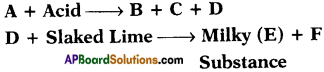If B is calcium chloride, what are A, C, D and E?
A is calcium carbonate or calcium hydrogen carbonate.
C is water and D is carbon dioxide.
E is calcium carbonate and F is water.

Question 23.
Some salts are given below. Classify them into hydrous and anhydrous salts. Sodium carbonate, Sodium chloride, Sodium hydrogen carbonate, Copper sulphate, Hypo, Magnesium Sulphate (epsum salt)
Hydrous salts :

1. Hypo (Na2S2O3 • 2H2O)
2. Epsum (MgSO4 • 7H2O)
3. Copper sulphate (CuSO4 • 5H2O)

Anhydrous salts :

1. Sodium chloride (NaCl)
2. Sodium carbonate (Na2CO3)
3. Sodium hydrogen Carbonate (NaHCO3)

Question 24.
If someone in the family is suffering from a problem of acidity, which of the following would you suggest as a remedy : lemon juice, vinegar or baking soda solution? Which property do you think of while suggesting the remedy?

• I suggest baking soda solution. As acidity can be neutralized by baking soda solution, we can use it.
• Neutralizing property of baking soda solution.

Question 25.
Why are curd and sour substances not kept in copper vessels?
Curd and sour substances contain acids which react with copper vessels and form poisonous substances. So curd and sour substances should not be kept in copper vessels.

Question 26.
Which gas is liberated when acids react with metals? Give one example.
When acids react with active metals they release hydrogen gas.
Zn(s) + 2HCl(aq) → Zncl2(aq) + H2(g)

Question 27.
Why should pickles not be stored in metallic containers?
Pickles contain acids which react with metallic containers and form poisonous substance. So they are kept in plastic containers.Question 28.
Solution x turned blue litmus red and Solution y turned red litmus blue.
a) What products could be formed when x and y are mixed?
b) Which gas is released when we put magnesium pieces in solution x?
c) Will any chemical reaction take place when zinc pieces are put in solution y?
d) Which of the above solutions contain more hydrogen ions?
Given solution Y turned blue litmus into red so, Y is an acid.
Given solution ‘y’ turned red litmus into blue so, ‘y’ is a base.
a) The reaction of an acid (x) with a base to give a salt and water.
b) When we put magnesium pieces in solution releases hydrogen gas.
c) When zinc pieces are put in solution y, a chemical reaction will take place there.
d) Acids contain more H+ ions in the given solutions, Y has more H+ ions because it is an acid.

Question 29.
Acid should be added to water but not water to the acid. Why?

• The dissolving of an acid or base in water is an highly exothermic process. Care must be taken while mixing concentrated HNO3 or concentrated H2SO4 with water.
• The acid must always be added slowly to water with constant stirring.
• If water is added to a concentrated acid, the heat generated may cause the mixture to splash out and cause bums.
• The glass container may also break due to excessive local heating.

Question 30.
Explain the procedure to confirm the given salt is a hydrous or anhydrous.

1. Take given salt in a test tube
2. Observe the colour of salt
3. Heat the test tube gently
4. Observe the colour of salt and also moisture (droplets) inside of the test tube walls.
5. If its colour changes or forms water droplets, it is hydrous salt.
6. Otherwise, it is anhydrous salt.

Question 31.
Categorize the following as acids, bases, and salts :
Lemon juice, salt water, soap water, tamarind juice, surf water, lime water.
Acids :

1. Lemon juice
2. Tamarind juice

Bases :

1. Soap water
2. Surf water
3. Lime water

Salts :

1. Salt water

Question 32.
Classify the following salts as family of salts having same cation or anion and prepare a table.
Potassium sulphate, Sodium sulphate, Calcium sulphate, Magnesium sulphate, Copper sulphate, Sodium chloride, Sodium nitrate, Sodium carbonate and Ammonium chloride.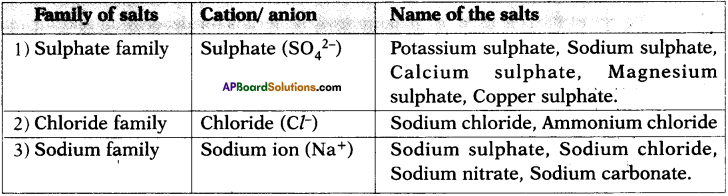Question 33.
Observe the table and answer the following questions.

 Solutions pH value Blood 7.3 Pure water 7.0 Gastric fluid 1.2 Sodium hydroxide 13

1) Which of the solutions among these is a strongest base?
2) Which body fluid has slightly basic nature?
3) What is the nature of pure water?
4) Which body fluid is strongest acid?

1. Sodium hydroxide because its pH is 13.
2. Blood because its pH is 7.3.
3. Pure water is neutral in nature because its pH is 7.
4. Gastric juice because its pH is 1.2.

Question 34.
The diagram given below shows the removal of water crystallisation. Find error in the diagram.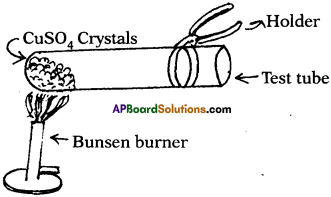Error in the diagram

1. Test tube is placed towards observer. It causes bums on his hands.
2. So, test tube should be placed away from the observer.

Question 35.
What are the uses of Plaster of Paris?

• The substance which doctors use as plaster for supporting fractured bones in the right position.
• Plaster of Paris is used for making toys, materials for decoration and for making surfaces smooth.Question 36.
What are the applications of pH in daily life?
1) In medical science :
The pH values of urine and blood are taken for diagnosis of various diseases.

2) In dairies :
Milk has pH of 6.6. A change in the pH of milk indicates that milk has turned sour.

3) In agriculture :
For better growth of crops the pH of the soil is regularly tested.
For examples :

1. Citrus fruits require slightly alkaline soil.
2. Rice requires acidic medium.
3. Sugarcane requires neutral soil.

4) In technology:
Organic and biochemical reactions are carried out under control pH.

### 10th Class Chemistry 4th Lesson Acids, Bases and Salts 4 Marks Important Questions and Answers

Question 1.
Draw a neat diagram showing a base solution in water conducts electricity. Why the solution of sugar/glucose in water do not conduct electricity? (AP March 2017)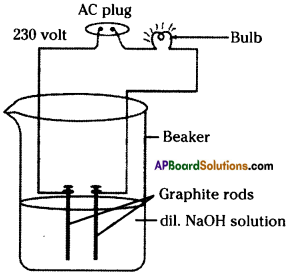The solution of sugar/glucose in water do not conduct electricity because there is no H+ ions in the solution.

Question 2.
Explain an activity to show the water of crystallisation in CuSO4 • 5H2O. (AP June 2018)

• Take a few crystals of copper sulphate in a dry test tube and heat the test tube.
• We observe water droplets on the walls of the test tube and salt turns white.
• Add 2 – 3 drops of water on the sample of copper sulphate obtained after heating.
• We observe the blue colour of copper sulphate crystals is restored.

Question 3.
Read the information given in the table and answer the following questions. (TS March 2016)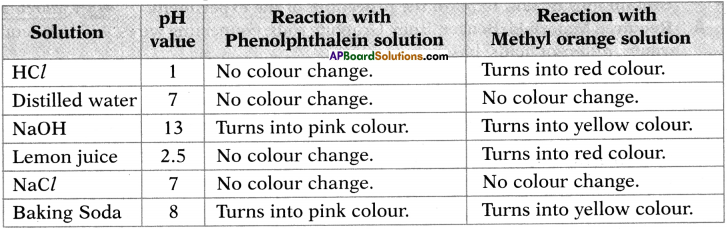a) List out the acids in the above table.
The acids are HCl and lemon juice.

b) What is the nature of the solution which gives pink colour with Phenolphthalene solution?
The nature of the solution which turns pink colour with phenolphthalene solution is basic.

c) List out the neutral solutions in the above table.
The neutral solutions are distilled water and NaCl.

d) Name the strongest acid and the strongest base among the given solutions.
The strongest acid is HCl and the strongest base is NaOH.

Question 4.
Observe the following table and answer the questions given below. (TS June 2o17)
The table contains the aqueous solutions of different substances with the same concentrations and their respective pH values.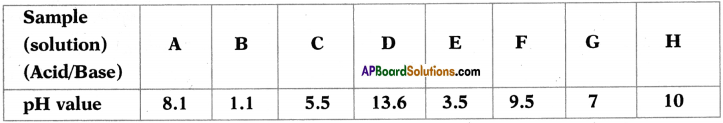i) Which one of the above acid solutions is the weakest acid? Give a reason.
Weakest acid is ‘C’. Because its pH value is less than 7 and it is nearer to 7.

ii) Which one of the above solutions is the strongest base? Give a reason.
Strongest base is ‘D’. Because it’s pH value is near to 14.

iii) Which of the above two produce maximum heat when they react ? What does that heat energy called?
B, D produce maximum heat when they react. This heat energy is known as neutralization energy.

iv) Which one of the above solutions has the pH equal to that of the distilled water? What is the name given to solutions of that pH value?
‘G’ has the pH equal to that of the distilled water. These type of solutions are known as neutral solution.

Question 5.
List out the materials required to test whether the solutions of given acids and bases contain ions or not. Explain the procedure of the experiment. (TS March 2017)
Required Materials :
Beaker, Bulb, Graphite rods, connecting wires, 230 V AC current, water, different acids, bases.

Experimental Procedure :

1. Connect the two connecting wires to the graphite rods.
2. Keep the graphite rods into the beaker, take care that two graphite rods do not touch each other.
3. Arrange a bulb in the circuit.
4. Pour dilute acid into the beaker.
5. Connect the ends of the connectors to 230 V AC.
6. In this way, change the acid / base and do the experiment.

The bulb glows in the experiment when the beaker contains acid or base. Hence, when the bulb glows we can say that acid or base contain ions.Question 6.
List out the material for the experiment “when Hydrochloric acid reacts with NaHCO3 and evolves CO2“. Write the experimental procedure. (TS March 2018)
Required material : Stand, test tubes, delivery tube, thistle funnel, two hole rubber corks, Ca(OH)2, NaHCO3, HCl.

Experimental procedure :

1. Take NaHCO3 in a test tube and fix two hole cork to the test tube.
2. Fix thistle funnel in one hole of cork and insert delivery tube in the second hole of the cork. Insert the second end of the delivery tube in the other test tube which is containing Ca(OH)2/lime water.
3. Pour dil. HCl into the test tube using thistle funnel.
4. Due to chemical reaction, gas is evolved and pass into the Ca(OH)2 through delivery tube. It turns in to milky. We can conclude that it is CO2 gas.

Question 7.
Prepare a table based on the colour responses of acid, base and salt with indicators such as indicators. (AP SA-I:2018-19)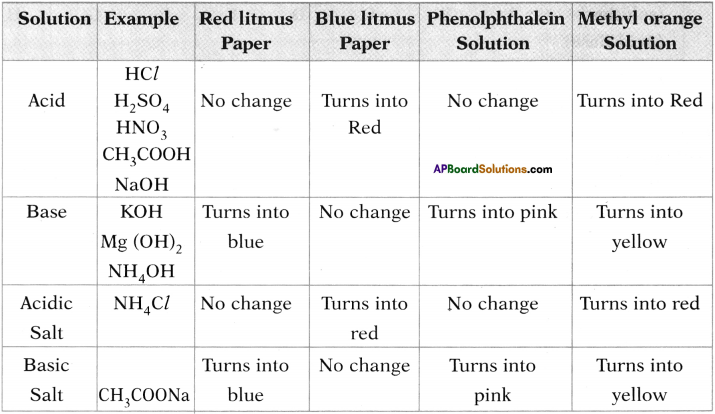Question 8.
Draw universal pH value indicator and identify different substances. (AP SA-I : 2019-20)
(OR)
Draw a neat diagram showing variation of pH with the chage in concentration of H+(aq) ion and OH(aq) ions.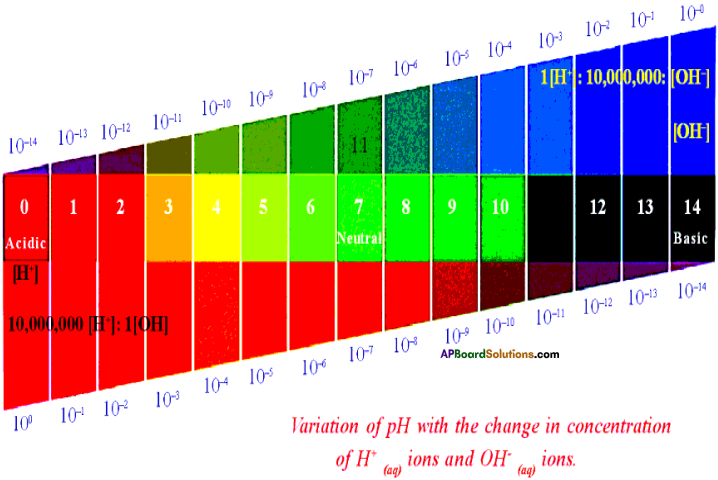Variation of pH with the change in concentration of H+(aq) ions and OH(aq) ions.

Question 9.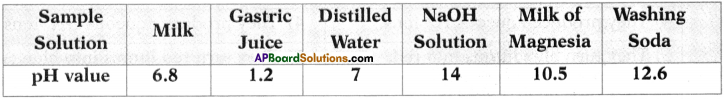Answer the following questions by using above information. (TS June 2019)
1) Which of the above is neutral solution?
2) Which of the above is used to neutralize the acidity in stomach?
3) Which is the strong acid among the above solutions?
4) What is the colour of Phenolphthalein indicator in NaOH solution?

1. Distilled water
2. Milk of Magnesia
3. Gastric juice
4. Pink

Question 10.
If the pH values of solutions X, Y and Z are 13, 6 and 2 respectively, then
a) Which solution is a strong acid? Why?
b) Which solution contains ions along with molecules of solution?
c) Which solution is a strong base? Why?
d) Does the pH value of a solution increase or decrease when a base is added to it? Why?
The strength of an acid (or) an alkali can be tested by using pH value of a solution. If the value of a pH of a solution is less, then that solution exhibits acidic nature.

If the value of a pH of a solution is more, then that solution exhibits basic nature.
pH value of a solution “X” is 13
pH value of a solution “Y” is 6
pH value of a solution “Z” is 2

a) Solution ‘Z’ is strong acid because its pH is 2.
b) Among given solutions, solution X is weakest acid. Weak solution contains ions along with molecules of solution. So X exhibits like this character.
c) Solution X is strong base. Because its pH is 13.
d) If base is added to solution ‘Z’, then its pH will increase.

Question 11.
Distinguish between acids and bases.

 Acids Bases 1) They are sour to taste. 1) They are better to taste and soapy to touch. 2) When non-metallic oxides dissolved in water they form acids. 2) When metallic oxides dissolved in water they form bases. 3) They react with bases to form salt and water. 3) They react with acids to form salt and water. 4) They produce aqueous H+ ions. 4) They produce aqueous OH- ions. 5) They turn blue litmus into red. 5) They turn red litmus into blue. 6) They turn methyl orange indicator to red. 6) They turn methyl orange indicator to yellow. 7) The turn phenolphthalein indicator to colourless. 7) They turn phenolphthalien indicator to pink.

Question 12.
Explain chlor-alkali process.
When electricity is passed through an aqueous solution of sodium chloride, it decomposes to form sodium hydroxide. The process is called chloralkali process because of the products formed chlor for chlorine and alkali for sodium hydroxide.
2 NaCl (aq) + 2H2O(l) → 2NaOH(aq) + Cl2(g) + H2(g)

Chlorine gas is given off at the anode and hydrogen gas at the cathode and sodium hydroxide is formed near the cathode.

Question 13.
Define the following. Give one example for each.
a) Strong acid
b) Strong base
c) Weak acid
d) Weak base.
a) Strong acid :
The acid which undergoes 100% ionisation is called strong acid.
e.g.: HCl, H2SO4

b) Strong base :
The base which undergoes 100% ionisation is called strong base.
e.g.: NaOH, KOH

c) Weak acid:
The acid which undergoes less than 100% ionisation is called weak acid.
e.g.: CH3COOH, H2CO3

d) Weak base:
The base which undergoes less than 100% ionisation is called weak base.
e.g.: NH4OH, Mg(OH)2Question 14.
Write any four chemical properties of acids.
Chemical properties of acids :
1) Active metals react with acids and liberate hydrogen gas.
Zn + HCl → ZnCl2 + H2

2) Acids react with bases to form salt and water.
HCl(aq) + NaOH(aq) → NaCl(aq) + H2O(l)

3) Acids react with metallic oxides to form salt and water.
MgO(s)+ 2HCl(aq) → MgCl2(aq) + H2O(l)

4) Acids react with carbonates and hydrogen carbonates and release carbon dioxide gas.
CaCO3(s) + 2HCl(aq) → CaCl2(aq)+ H2O(l) + CO2(g)
Ca(HCO3)2(l) + 2HCl(aq) → CaCl2(aq) + 2H20((| + 2CO2(g)

Question 15.
Write the formulae of the following salts.
a) Sodium sulphate
b) Ammonium chloride
Identify the acids and bases for which the above salts are obtained. Also write chemical equations for the reactions between such acids and bases. Which type of chemical reactions are they?
a) Formula of sodium sulphate is Na2S04. When sulphuric acid reacts with sodium hydroxide it forms sodium sulphate.
H2SO4(aq)+ 2NaOH(aq) → Na2SO4(aq) + 2H2O(l)

b) Formula of Ammonium chloride is NH4C/. When Ammonium hydroxide reacts with hydrochloric acid it forms Ammonium chloride.
NH4OH(aq) + HCl(aq) → NH4Cl(aq) + H2O(l)

Question 16.
Write balanced equations to satisfy each statement,
a) Acid + Active metal → Salt + Hydrogen
Zn(s) + 2HCl(aq) → ZnCl2(aq) + H2(g)

b) Acid + Base → Salt + Water
NaOH(aq) + HCl(aq) → NaCl(aq) + H2O(l)

c) Acid + Carbonate / Hydrogen carbonate → Salt + Water + Carbon dioxide
CaCO3(s) + 2 HCl(aq) → CaCl2(aq) + H2O(l) + CO2(g)
NaHCO3(s) + HCl(aq) → NaCl(aq) + H2O(l) + CO2(g)

d) Metal oxide + Acid → Salt + Water
CaO(s) + 2 HCl(aq) → CaCl2(aq) + H(2)O(l)

e) Non metal oxide + base → Salt + Water
CO2(g) + 2 NaOH(aq) → Na2CO3(aq) + H2O(l)

Question 17.
Give important products obtained from chloralkali process.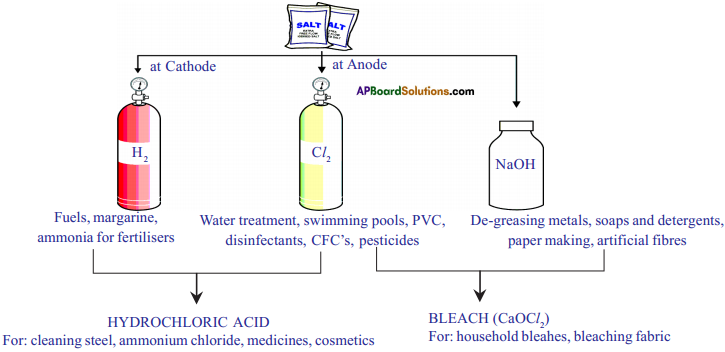Result:
Five water molecules are present in one formula unit of copper sulphate. Water of crystallization proves that the crystals contain a fixed quantity of water in them.

Question 18.
Give the equations for the preparation of each of the following.
i) Copper sulphate from copper (II) oxide.
CuO + H2SO4 → CuSO4 + H2O

ii) Potassium sulphate from potassium hydroxide solution.
2 KOH + H2SO4 → K2SO4 + 2 H2O

PbCO3 + 2 HCl → PbCl2 + H2O + CO2

Question 19.
How are the following salts prepared?
1) Calcium sulphate from calcium carbonate
When calcium carbonate is treated with sulphuric acid it forms calcium sulphate.
CaCO3 + H2SO4 → CaSO4 + H2O + CO2

When lead nitrate is treated with carbonic acid we will get lead carbonate.
Pb(NO3)2 + H2CO3 → PbCO3 + 2 HNO3

3) Sodium nitrate from sodium hydroxide
When sodium hydroxide is reacted with nitric acid it will form sodium nitrate.
NaOH + HNO3 → NaNO3 + H2O

4) Magnesium carbonate from magnesium chloride
When magnesium carbonate is reacted with hydrochloric acid it forms magnesium chloride.
MgCO3 + 2 HCl → MgCl2 + H2O + CO2Question 20.
Which of the following reactions are considered as neutralization reactions? Why?
1) NaOH + HCl → NaCl + H2O
2) CaO + 2 HCl → CaCl2 + H2O
3) CO2 + 2 NaOH → Na2CO3 + H2O
4) SiO2 + CaO → CaSiO3
All of them are considered as neutralization reactions.

1. An acid (HCl) reacts with base (NaOH) and forms salt and water. So it is a neutralization reaction.
2. Here metallic oxide which is basic in nature reacts with acid and forms salt and water. So it is also a neutralization reaction.
3. In third case non-metallic oxide (acidic oxide) reacts with base (NaOH) and forms salt and water. So it is also a neutralization reaction.
4. In fourth case a metallic oxide (CaO) reacts with non-metallic oxide (SiO2) and forms salt. So it is also a neutralization reaction in the absence 6f water.

Question 21.
Which metals produce hydrogen gas when they are reacted with bases like NaOH and KOH? Write the chemical equations for the reactions.
Zinc, aluminium and lead react with bases like NaOH and KOH and produce hydrogen gas.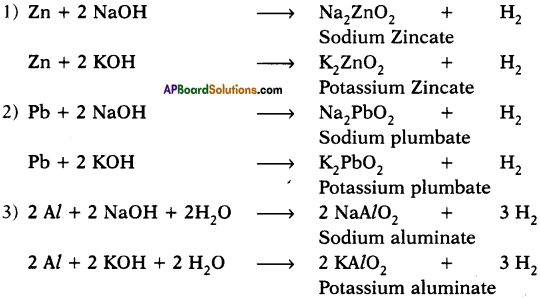Question 22.
i) A solution has a pH of 7. How would you increase its pH and decrease its pH? Explain.
We can increase the pH of a solution by adding base because we know that bases have pH > 7. We can decrease the pH of a solution by adding an acid because acidic solution have pH < 7.

ii) If a solution changes the colour of litmus from red to blue, then what can you say about its pH?
Bases can change red litmus into blue. So the pH of the solution is greater than 7.

iii) What can you say about pH of a solution that liberates carbon dioxide from sodium carbonate?
Acids react with carbonates and liberate hydrogen gas. So the pH of the solution is less than 7.

Question 23.
Write the pH values of some solutions.

 pH value Solutions 0 Battery Acid 1 Con. H2S04 2 Lemon juice 3 Orange juice 4 Tomato juice 5 Black coffee, Bananas 6 Milk, urine 7 Pure water 8 Sea water, eggs 9 Baking soda 10 Milk of magnesia 11 Ammonia solution 12 Soapy water 13 Bleach oven cleaner 14 Liquid drain cleaner

Question 24.
Fill the following table of results of reactions between some substances (acids, bases, neutral substances) and indicators.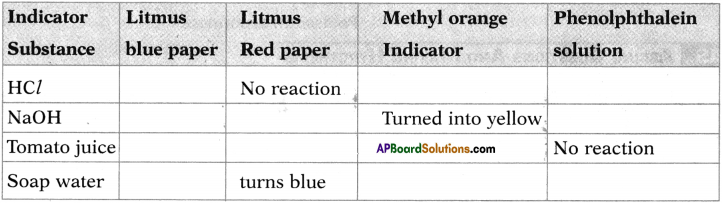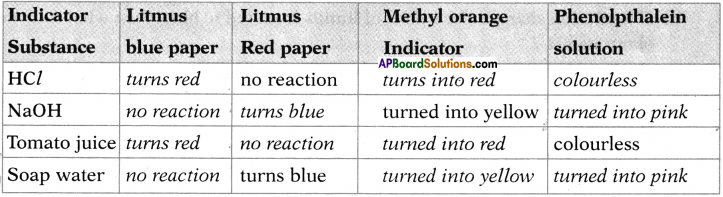Question 25.
The pH values of six solutions A, B, C, D, E, F are given as 5,2,1,3,7 and 9 respectively. Which solution is
a) Neutral
b) Strongly alkaline
c) Strongly acidic
d) Weakly acidic?
Arrange the pH in increasing order of Hydrogen ion concentration.
a) Solution E is neutral.
b) Solution F is Alkaline.
c) Solution C is strongly acidic.
d) Solution A is weakly acidic.
e) Solution B is strongly acidic.
f) Solution D is strongly acidic.
g) Ascending order of increase of Hydrogen ion concentration is F, E, A, D, B, C.

Question 26.
Collect information about various organic acids different occurring naturally and prepare a table.

 1. Acetic acid Vinegar (obtained from fruits after fermentation). 2. Citric acid Citrus fruits like orange and lemons. 3. Butyric acid Butter gone bad or rancid 4. Lactic acid Curd 5. Malic acid Apples 6. Oleic acid Olive oil 7. Tartaric acid Fruits such as grapes, apples and tamarind 8. Stearic acid From fats 9. Succinic acid From vegetables like lettuce and unripe fruits 10. Uric acid From urine

Question 27.
Complete the table.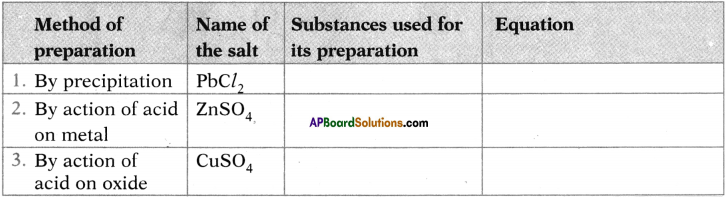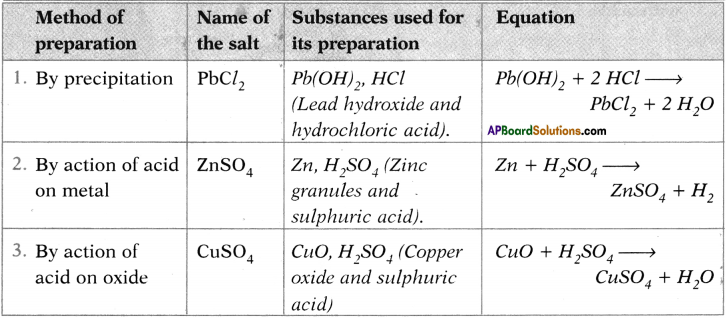Question 28.
Fill the table.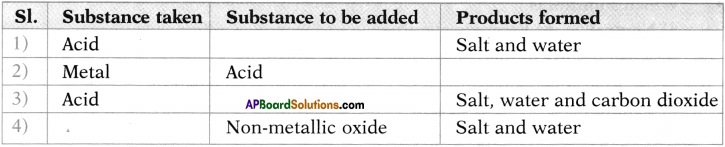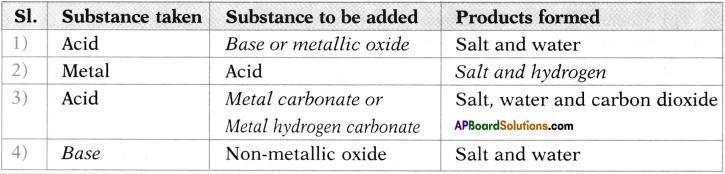Question 29.
Draw a diagram to show the reaction of acids with metals.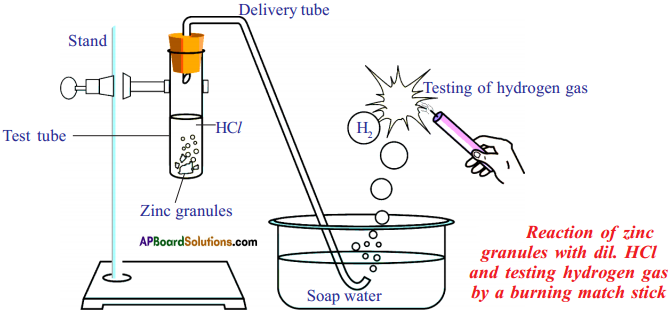Reaction of Zinc granules with dil. HCl and testing hydrogen gas by a burning candle

Question 30.
Draw a diagram to show that all metal carbonates and react hydrogen carbonates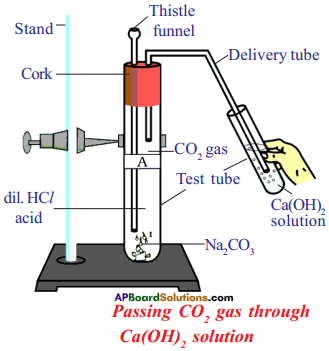Question 31.
What are the uses of Bleaching powder?

• It is used for bleaching cotton and linen in the textile industry for bleaching wood pulp in paper industry and for bleaching washed clothes in laundry.
• Used as an oxidizing agent in many chemical industries.
• Used for disinfecting drinking water to make it free of germs.
• Used as a reagent in the preparation of chloroform.

Question 32.
What are the uses of Baking soda?
1) Baking powder is a mixture of baking soda and a mild edible acid such as tartaric acid. When baking powder is heated or mixed in water, the following reaction takes place.

Carbon dioxide produced during the reaction causes bread or cake to rise making them soft and spongy.

2) Sodium hydrogen carbonate is also an ingredient in antacids. Being alkaline, it neutralizes excess acid in the stomach and provides relief.

3) It is also used in soda-acid, fire extinguishers.

4) It acts as mild antiseptic.

Question 33.
What are the uses of Washing soda?

• Sodium carbonate (washing soda) is used in glass, soaps and paper industries.
• It is used in the manufacture of sodium compounds such as borax.
• Sodium carbonate can be used” as a cleaning agent for domestic purposes.
• It is used for removing permanent hardness of water.Question 34.
Write the chemical formulae of the following :
i) Bleaching powder
ii) Sodium Chloride
iii) Slaked lime
iv) Baking Soda
v) Washing Soda
vi) Gypsum
vii) Plaster of Paris
viii) Acetic acid
ix) Sodium Hydroxide
x) Limestone
i) Bleaching powder = CaOCl2
ii) Sodium Chloride = NaCl (Common Salt)
iii) Slaked lime (or) lime water = Ca(OH)2
iv) Baking Soda = NaHCO3
v) Washing Soda = Na2CO3, 10H2O
vi) Gypsum = CaSO4 . 2H2O
vii) Plaster of Paris = CaSO4 . ½H2O
viii) Acetic acid = CH3COOH
ix) Sodium Hydroxide = NaOH
x) Limestone = CaCO3

Question 35.
What are the various applications of neutralization?

• The acidity of soil is reduced by adding slaked lime.
• The sting of yellow wasps contains alkalis. If acetic acid is rubbed on affected area, they are neutralized.
• Ants and bees have formic acid in their stings which can be neutralised by applying soap and some other alkali.
• Antacids tablets contain magnesium hydroxide, persons suffering from acidity are administered these tablets.
• The affect of nettle plant leaves is neutralized by leaves of dock plant.Question 36.
Mention two situations where you use hydrated and unhydrated salts in your daily life.